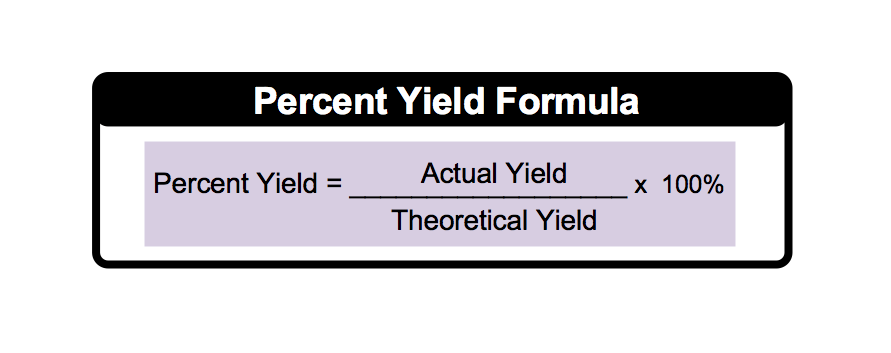Clutch Prep is now a part of Pearson
Ch.3 - Chemical ReactionsWorksheetSee all chapters

# Percent Yield

See all sections
Sections
Empirical Formula
Molecular Formula
Combustion Analysis
Combustion Apparatus
Polyatomic Ions
Naming Ionic Compounds
Writing Ionic Compounds
Naming Ionic Hydrates
Naming Acids
Naming Molecular Compounds
Balancing Chemical Equations
Stoichiometry
Limiting Reagent
Percent Yield
Mass Percent
Functional Groups in Chemistry

The Percent Yield determines how successful the product yield is in a chemical reaction.

###### Percent Yield

Concept #1: Percent YieldExample #1: Consider the following balanced chemical reaction:
2 C6H6 (l) + 15 O2 (g) → 12 CO2 (g) + 6 H2O (l)

If a 2.6 g sample of C6H6 reacted with excess O2 to produce 1.25 g of water, what is the percent yield of water?

Practice: Ammonia, NH3, reacts with hypochlorite ion, OCl, to produce hydrazine, N2H4. How many grams of hydrazine are produced from 115.0 g NH3 if the reaction has a 81.5% yield?

2 NH3 + OCl → N24 + Cl + H2O

Practice: The reduction of iron (III) oxide creates the following reaction:

Fe2O3 (s) + 3 H2 (g) → 2 Fe (s) + 3 H2O (g)

If the above reaction only went to 75% completion, how many moles of Fe2O3 were require to produce 0.850 moles of Fe?